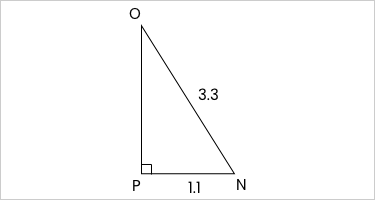### Still have math questions?

Trigonometry
Question

In $$\triangle\text{NOP}$$, the measure of $$\angle\text{P}=90^{\circ}$$$$\text{PN}=1.1$$, and $$\text{NO}=3.3$$ feet. Find the measure of $$\angle\text{N}$$ to the nearest degree.$$\angle\text{N}=71^{\circ}$$